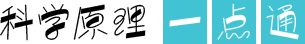# 趣味冰雹猜想

2019-07-26 09:00  来源： 新华网9×3+1=28,28÷2=14，14÷2=7，7×3+1=22，22÷2=11，11×3+1=34，34÷2=17，17×3+1=52，52÷2=26，26÷2=13，13×3+1=40，40÷2=20，20÷2=10，10÷2=5，5×3+1=16，16÷2=8，8÷2=4，4÷2=2，2÷2=1。

① 1→2→1；

② 5→14→7→20→10→5；

③ 17→50→25→74→37→110→55→164→82→41→122→61→182→91→272→136→68→34→17。• 1

• 2

• 3

• 4

• 5